• 问答
• 技术
• 实践
• 资源

## 一、前言

Transformer 可以做很多有趣而又有意义的事情。

AI 生成的图像：Transformer 最初主要应用于一些自然语言处理场景，比如翻译、文本分类、写小说、写歌等。Transformer 近两年非常火爆，内容也很多，要想讲清楚，还涉及一些基于该结构的预训练模型，例如著名的 BERTGPT，以及刚出的 DALL·E 等。

## 二、Transformer

TransformerGoogle2017 年提出的用于机器翻译的模型。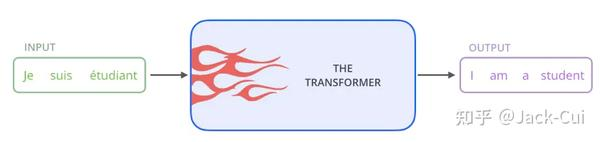Transformer 的内部，在本质上是一个 Encoder-Decoder 的结构，即 编码器-解码器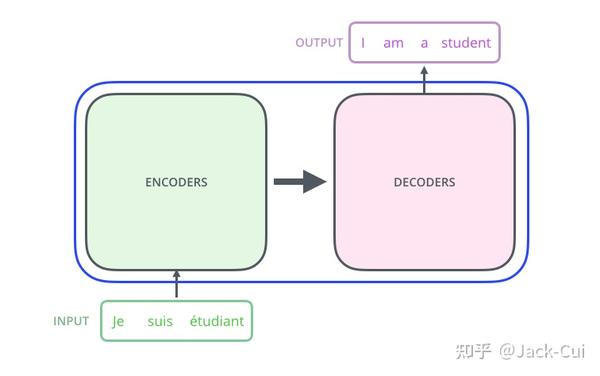Transformer 中抛弃了传统的 CNNRNN，整个网络结构完全由 Attention 机制组成，并且采用了 6Encoder-Decoder 结构。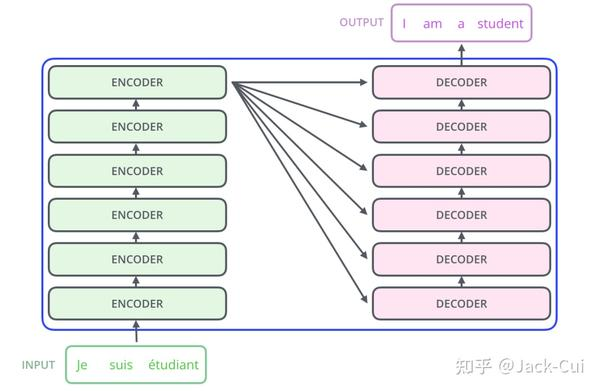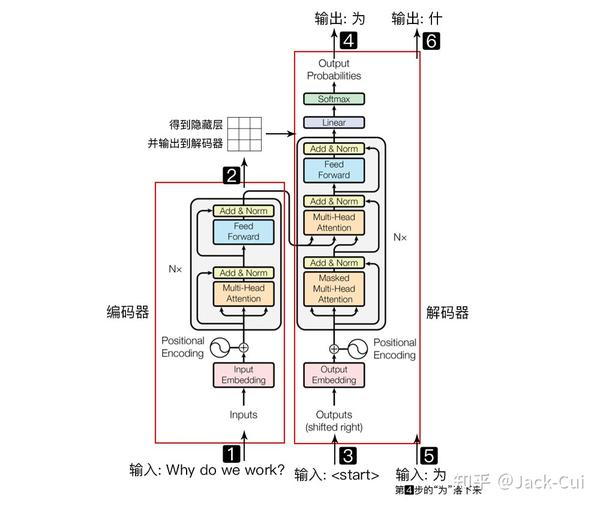Why do we work?，我们为什么工作？

• 输入自然语言序列到编码器: Why do we work\?(为什么要工作)；
• 编码器输出的隐藏层，再输入到解码器；
• 输入 \< > (起始)符号到解码器；
• 解码器得到第一个字"为"；
• 将得到的第一个字"为"落下来再输入到解码器；
• 解码器得到第二个字"什"；
• 将得到的第二字再落下来，直到解码器输出 \< > (终止符)，即序列生成完成。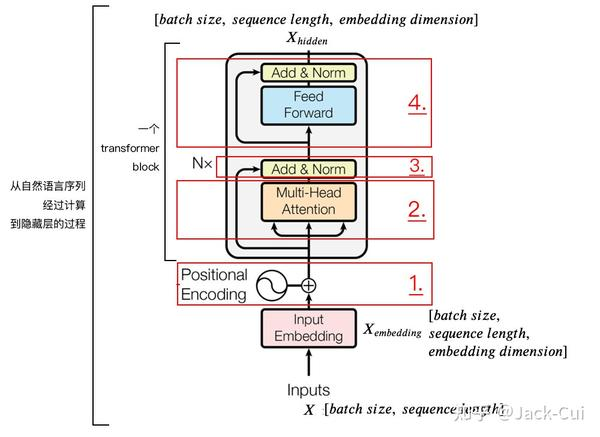### 1、位置嵌入（ ）

batch size 就是 batch 的大小，这里只有一句话，所以 batch size1sequence length 是句子的长度，一共 7 个字，所以输入的数据维度是 [1, 7]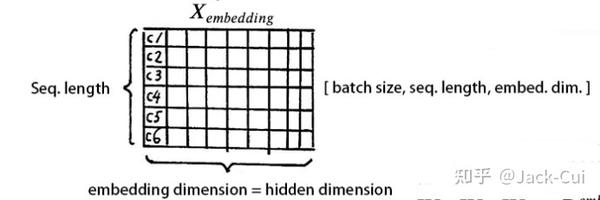Tranformer 采用的是 sin-cos 规则，使用了 sincos 函数的线性变换来提供给模型位置信息：

\begin{aligned} P E{(p o s, 2 i)} &=\sin \left(p o s / 10000^{2 i / d{\text {model }}}\right) \ P E{(\text {pos }, 2 i+1)} &=\cos \left(\text { pos } / 10000^{2 i / d{\text {model }}}\right) \end{aligned}

# 导入依赖库
import numpy as np
import matplotlib.pyplot as plt
import seaborn as sns
import math

def get_positional_encoding(max_seq_len, embed_dim):
# 初始化一个positional encoding
# embed_dim: 字嵌入的维度
# max_seq_len: 最大的序列长度
positional_encoding = np.array([
[pos / np.power(10000, 2 * i / embed_dim) for i in range(embed_dim)]
if pos != 0 else np.zeros(embed_dim) for pos in range(max_seq_len)])
positional_encoding[1:, 0::2] = np.sin(positional_encoding[1:, 0::2])  # dim 2i 偶数
positional_encoding[1:, 1::2] = np.cos(positional_encoding[1:, 1::2])  # dim 2i+1 奇数
# 归一化, 用位置嵌入的每一行除以它的模长
# denominator = np.sqrt(np.sum(position_enc**2, axis=1, keepdims=True))
# position_enc = position_enc / (denominator + 1e-8)
return positional_encoding

positional_encoding = get_positional_encoding(max_seq_len=100, embed_dim=16)
plt.figure(figsize=(10,10))
sns.heatmap(positional_encoding)
plt.title("Sinusoidal Function")
plt.xlabel("hidden dimension")
plt.ylabel("sequence length")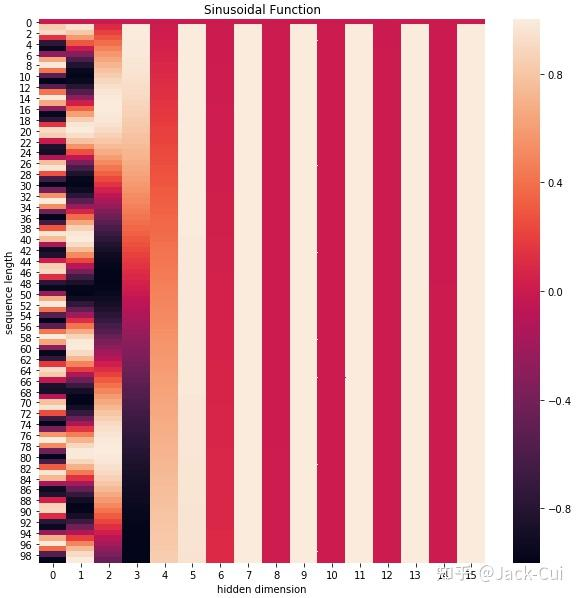### 2、自注意力层（ ℎ ）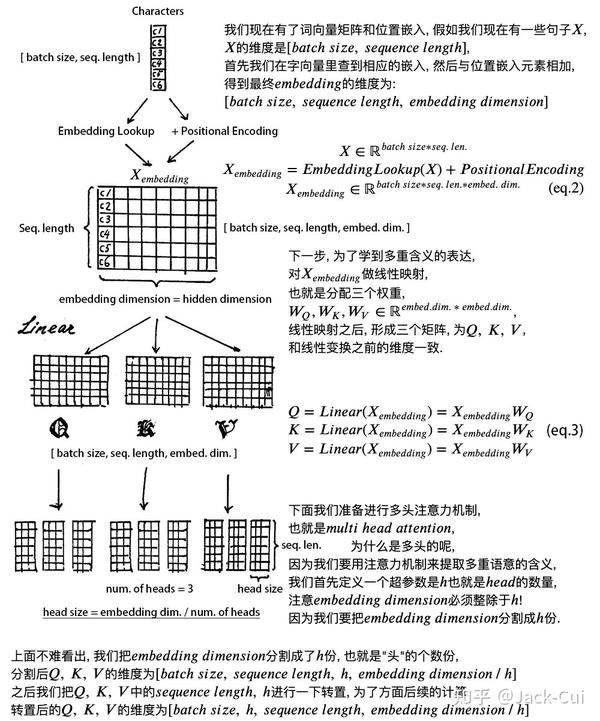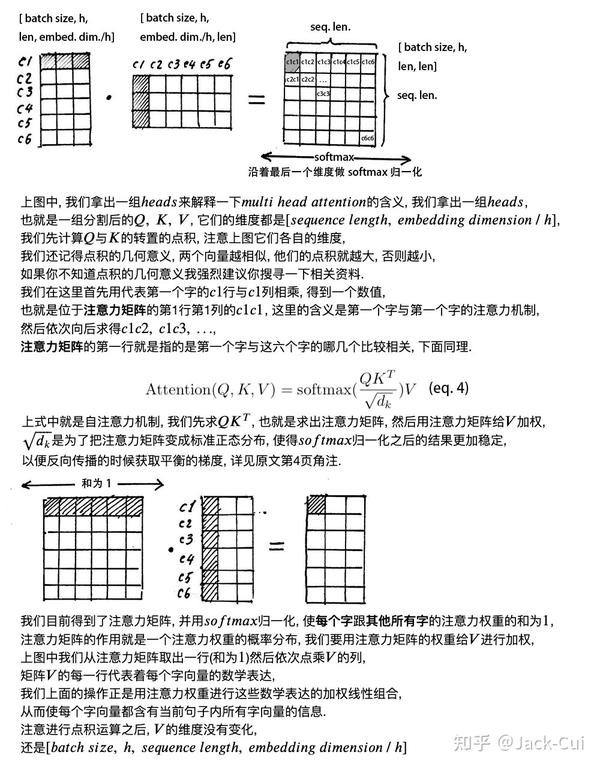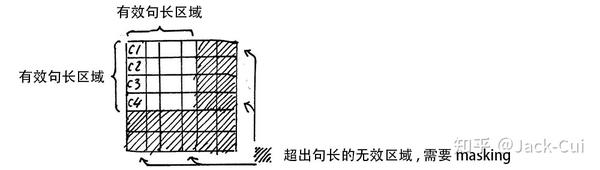### 1) 残差设计

$$X_{embedding} + Attention(Q, \ K, \ V) \$$

$$X + SubLayer(X) \$$

### 2) 层归一化

$$\mu{i}=\frac{1}{m} \sum^{m}{i=1}x_{ij} \$$

$$\sigma^{2}{j}=\frac{1}{m} \sum^{m}{i=1} (x{ij}-\mu{j})^{2}\$$

$$LayerNorm(x)=\alpha \odot \frac{x{ij}-\mu{i}} {\sqrt{\sigma^{2}_{i}+\epsilon}} + \beta \$$

class ScaledDotProductAttention(nn.Module):
''' Scaled Dot-Product Attention '''

def __init__(self, temperature, attn_dropout=0.1):
super().__init__()
self.temperature = temperature
self.dropout = nn.Dropout(attn_dropout)

def forward(self, q, k, v, mask=None):
# self.temperature是论文中的d_k ** 0.5，防止梯度过大
# QxK/sqrt(dk)
attn = torch.matmul(q / self.temperature, k.transpose(2, 3))

if mask is not None:
# 屏蔽不想要的输出
attn = attn.masked_fill(mask == 0, -1e9)
# softmax+dropout
attn = self.dropout(F.softmax(attn, dim=-1))
# 概率分布xV
output = torch.matmul(attn, v)

return output, attn

Multi-Head Attention 实现在 ScaledDotProductAttention 基础上构建：

class MultiHeadAttention(nn.Module):
''' Multi-Head Attention module '''

# n_head头的个数，默认是8
# d_model编码向量长度，例如本文说的512
# d_k, d_v的值一般会设置为 n_head * d_k=d_model，
# 此时concat后正好和原始输入一样，当然不相同也可以，因为后面有fc层
# 相当于将可学习矩阵分成独立的n_head份
def __init__(self, n_head, d_model, d_k, d_v, dropout=0.1):
super().__init__()
# 假设n_head=8，d_k=64
self.n_head = n_head
self.d_k = d_k
self.d_v = d_v
# d_model输入向量，n_head * d_k输出向量
# 可学习W^Q，W^K,W^V矩阵参数初始化
self.w_qs = nn.Linear(d_model, n_head * d_k, bias=False)
self.w_ks = nn.Linear(d_model, n_head * d_k, bias=False)
self.w_vs = nn.Linear(d_model, n_head * d_v, bias=False)
# 最后的输出维度变换操作
self.fc = nn.Linear(n_head * d_v, d_model, bias=False)
# 单头自注意力
self.attention = ScaledDotProductAttention(temperature=d_k ** 0.5)
self.dropout = nn.Dropout(dropout)
# 层归一化
self.layer_norm = nn.LayerNorm(d_model, eps=1e-6)

def forward(self, q, k, v, mask=None):
# 假设qkv输入是(b,100,512),100是训练每个样本最大单词个数
# 一般qkv相等，即自注意力
residual = q
# 将输入x和可学习矩阵相乘，得到(b,100,512)输出
# 其中512的含义其实是8x64，8个head，每个head的可学习矩阵为64维度
# q的输出是(b,100,8,64),kv也是一样
q = self.w_qs(q).view(sz_b, len_q, n_head, d_k)
k = self.w_ks(k).view(sz_b, len_k, n_head, d_k)
v = self.w_vs(v).view(sz_b, len_v, n_head, d_v)

# 变成(b,8,100,64)，方便后面计算，也就是8个头单独计算
q, k, v = q.transpose(1, 2), k.transpose(1, 2), v.transpose(1, 2)

if mask is not None:
mask = mask.unsqueeze(1)   # For head axis broadcasting.
# 输出q是(b,8,100,64),维持不变,内部计算流程是：
# q*k转置，除以d_k ** 0.5，输出维度是b,8,100,100即单词和单词直接的相似性
# 对最后一个维度进行softmax操作得到b,8,100,100
# 最后乘上V，得到b,8,100,64输出
q, attn = self.attention(q, k, v, mask=mask)

# b,100,8,64-->b,100,512
q = q.transpose(1, 2).contiguous().view(sz_b, len_q, -1)
q = self.dropout(self.fc(q))
# 残差计算
q += residual
# 层归一化，在512维度计算均值和方差，进行层归一化
q = self.layer_norm(q)

return q, attn

### 4、前馈网络

class PositionwiseFeedForward(nn.Module):
''' A two-feed-forward-layer module '''

def __init__(self, d_in, d_hid, dropout=0.1):
super().__init__()
# 两个fc层，对最后的512维度进行变换
self.w_1 = nn.Linear(d_in, d_hid) # position-wise
self.w_2 = nn.Linear(d_hid, d_in) # position-wise
self.layer_norm = nn.LayerNorm(d_in, eps=1e-6)
self.dropout = nn.Dropout(dropout)

def forward(self, x):
residual = x

x = self.w_2(F.relu(self.w_1(x)))
x = self.dropout(x)
x += residual

x = self.layer_norm(x)

return x

## 三、絮叨

\@Jack-Cui

----------手动分割线----------

Jack-Cui：我是如何成为算法工程师的，超详细的学习路线​zhuanlan.zhihu.comJack-Cui：看完谷歌师兄的刷题笔记，多语言通吃，秒杀 88\% Leetcode 题目​zhuanlan.zhihu.com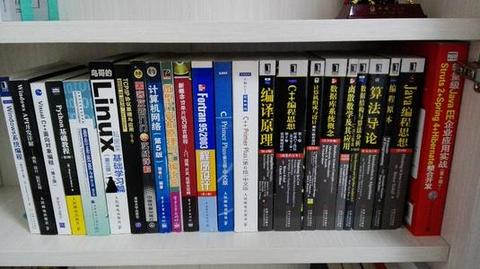•6
•0
•2413收藏

• 30

关注
• 70

获赞
• 17

精选文章

• 医学图像 图像分类

• 黑人的专栏

• 967
• 924
• 781
• 860
• 1.2k